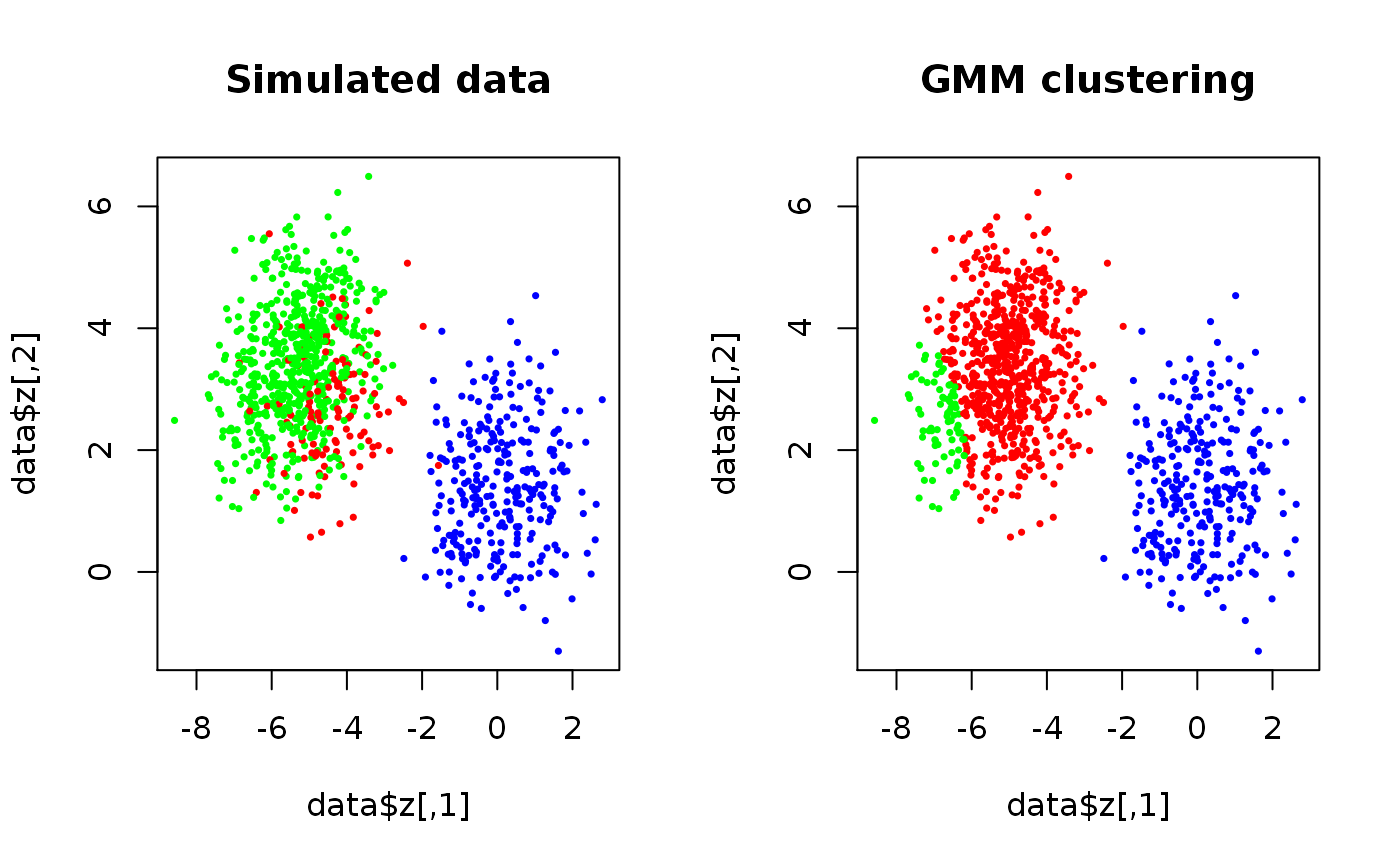The regular expectation-maximization algorithm for general multivariate Gaussian mixture models.

EMAlgorithm(x, theta, m, eps = 1e-06, max.ite = 1e+05,
trace.theta = FALSE, verbose = FALSE)

## Arguments

x A matrix of observations where each row correspond to an observation and each columns to a feature/variable. A list of parameters of class theta as described in rtheta. Optional. If not provided m should be given. numeric. The number of components if theta is not supplied. The maximal required difference in successive likelihoods to establish convergence. The maximum number of iterations. Logical. If TRUE, all estimates are stored and returned. Default is FALSE. Set to TRUE for verbose output. Default is FALSE.

## Value

A list of length 3 with elements:

theta

A list of the estimated parameters as described in rtheta.

loglik.tr

A numeric vector of the log-likelihood trace.

kappa

A matrix where kappa[i,j] is the probability that x[i, ] is realized from the j'th component.

## Details

Though not as versatile, the algorithm can be a faster alternative to Mclust in the mclust-package. If theta is not given, a k-means clustering is used to determine the initial theta.

rtheta, PseudoEMAlgorithm

## Author

Anders Ellern Bilgrau <anders.ellern.bilgrau@gmail.com>

## Examples

set.seed(3)
true.theta <- rtheta(d = 2, m = 3, method = "old")
true.theta$sigma <- lapply(true.theta$sigma, cov2cor) # Scale
if (FALSE) {
plot(true.theta, nlevels = 20, add.ellipses = TRUE)
}

data <- SimulateGMCMData(n = 1000, theta = true.theta)
start.theta <- rtheta(d = 2, m = 3)
start.theta$mu <- t(kmeans(data$z, 3)$centers) # More sensible location estimates start.theta <- as.theta(start.theta) # Coerce the matrix to a list res <- GMCM:::EMAlgorithm(data$z, theta = start.theta)

par(mfrow = c(1,2))
plot(data$z, cex = 0.5, pch = 16, main = "Simulated data", col = rainbow(3)[data$K])
plot(data$z, cex = 0.5, pch = 16, main = "GMM clustering", col = rainbow(3)[apply(res$kappa,1,which.max)])